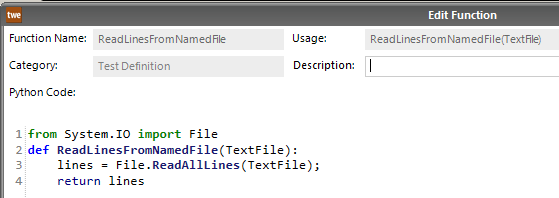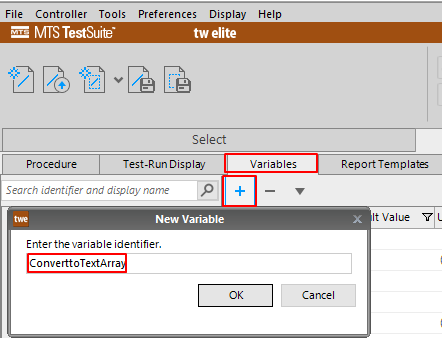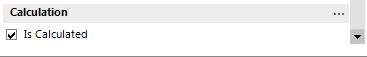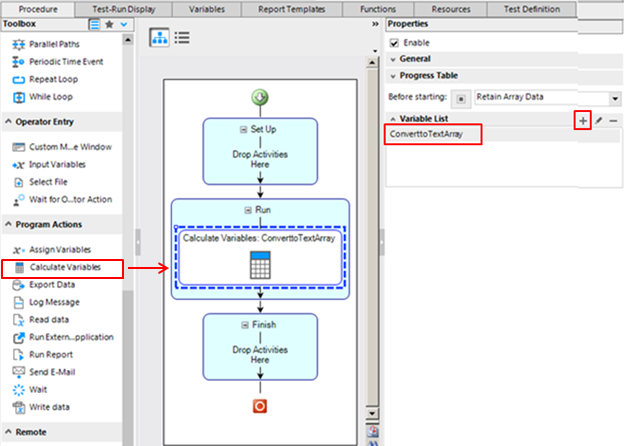# How are Python functions used in TestSuite?

QUESTION

How are Python functions used in TestSuite?

The programming languages of TestSuite

First it’s important to understand what programming languages TestSuite uses before explaining how they integrate with one another.

There are three different types of programming languages in TestSuite.

1. The visual programming language that allows the programmer to drag and drop test activities from the Toolbox to the TestFlow area.  This is an MTS proprietary software language.

2. The TestSuite written code used in the Calculations Editor.  This is an MTS proprietary software language.

3. The Python written code used in the Functions Editor window.  This is not an MTS proprietary language and is generally known to the programming public.

It is essential that the user first understand the visual programming language in order to layout the testflow.   After that, they must understand the written TestSuite code in order to write calculations for calculated variables.   The least important to know is Python.   Python should only be used when the user needs to perform a function in the Test that is not available with the existing functions in the software.

The user can then write functions using the Function Editor and call them up in the Calculations Editor under a calculated variable.

How the programming languages Integrate

The example below will demonstrate how the three programming languages integrate with one another.   Let’s say the user wants to read text from a text file into TestSuite.  This can be done by writing a function in Python that will read text from a text file and store it into a text array and pass it to a calculated variable using a Calculate Variables activity in the test   procedure.

Write a function using (Python witten code).

Use the plus sign (+) on the Functions tab to open the Edit Function window (Figure 1).Figure 1 – Opening the Edit Funtion Window to add a new function

Write a function using Python code called ReadLinesFromNamedFile (Figure 2).Figure 2 - Python Function in the TestSuite Function Editor to read text from text file

Create a new Text Variable using (TestSuite written code)

Click on the plus sign (+) on the Variable tab to open the New Variable window and enter the variable identifier, i.e., FileName (Figure 3).Figure 3 – Creating a new Variable named FileName

Click on the variable that was just created and open the Properties pane.

Select Text from the Type drop-down list, and next to Default Value, type the directory path to the text file, i.e., ‘C:\MyFolder\MtTextFile.txt’ (Figure 4).Figure 4 - Configuring the Properties for a Text variable

Create a new Calculated Text Array Variable using (TestSuite written code)

Click on the plus sign (+) on the Variable tab to open the New Variable window and enter the variable identifier, i.e., ConverttoTextArray (Figure 5).Figure 5 – Creating a new Variable named ConverttoTestArray

Click on the variable that was just created and open the Properties pane.  Check the Is Calculated check-box and select Text Array from the Type drop-down list (Figure 6).Figure 6 – Configuring the Properties for a Calculated Text Array variable

Click on the three dots () to open the Calculations Editor Window (Figure 7).Figure 7 – Link to the Calculations Editor Window

Click in the Calculation area and go to the Functions tab.  Scroll down and select the function ReadLinesFromNamedFile (Figure 8).  Highlight TextFile in the parenthesis.Figure 8 – Creating a Calculated variable from a Function

Click on the Variables tab and replace TextFile in the calculation with the text variable named File Name under the Variables list (Figure 9).Figure 9 – Validating the Calculation with the Parameter Variable FileName

Adding a Calculated Variable activity to the Test Procedure Editor using (TestSuite Visual Programming)

Click on the Procedure tab.  From the Toolbox, drag and drop a Calculate Variables activity to the Test Procedure Editor to where you want it in your test flow, and use the plus button (+) to select ConvertoTextArray from the Variables List (Figure 10).Figure 10 – Using a Calculate Variables activity in the Test Procedure to calculate the Calculated Variable ConvertoTextArray

K
Keary is the author of this solution article.

Did you find it helpful? Yes No

Send feedback